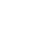# Looping with list comprehension

Sharing is caring!

Last Updated on July 24, 2022 by Jay

It’s hard to talk about Python without mentioning list comprehension, which is a looping technique in Python. The standard way to iterate (loop) through something is to use the `for .. in ..` statement. Looping with list comprehension is kind of like a syntax sugar, which looks like a for loop with a little twist to it. Sometimes list comprehension is referred to as “one line for loop”

This tutorial is part of the “Integrate Python with Excel” series, you can find the table of content here for easier navigation.

## For loop in Python

The standard way to loop is the for statement. The syntax is the following:

`for item in a_collection_of_items: do_something_with_item`

For example, let’s print each letter in the word “Python” using a `for` loop:

``````word = 'Python'
>>> for i in word:
print(i)

P
y
t
h
o
n``````

We’ll look at a second example – create a list of 10 numbers, from 0 to 9. We first created an empty list` li`, then loop through the 10 numbers and adding each one into the list.

``````li = []
for i in range(10):
li.append(i)

>>> li
[0, 1, 2, 3, 4, 5, 6, 7, 8, 9]``````

## List comprehension

The syntax of list comprehension kind of looks like a for loop. However, note the name “list” comprehension. What we are really doing is creating a list here. The syntax goes like the following, also note the square brackets which is used to represent a list:

`[do_something_with_item for item in a_collection_of_items]`

Let’s replicate the two for loop examples using list comprehension. Notice the last line of the code output: `[None, None, None, None, None, None]`. The for loop method didn’t return this line. What’s happening here?

``````word = 'Python'
>>> [print(i) for i in word]
P
y
t
h
o
n
[None, None, None, None, None, None]``````

Like I mentioned just earlier, list comprehension actually creates a list, thus the output line` [None, None, None, None, None, None]` is the list just got created. It’s a list of 6 None values. In Python, `print()` function doesn’t return any value, therefore “None”.

The second example might make more sense – we are creating a list of 10 items. Instead of the 3 lines of code using a for loop method, we can create a list using just 1 line, thus the name “one line for loop”.

``````li = [i for i in range(10)]
>>> li
[0, 1, 2, 3, 4, 5, 6, 7, 8, 9]``````

## Simple if conditional list comprehension

That’s correct, we can add logic conditions in a list comprehension. We’ll look at two different syntax here.

Syntax:

`[item for item in a_collection_of_items if condition]`

is equivalent to:

`for item in a_collection_of_items: if condition: do_something_with_item`

Let’s find all even numbers from the numbers 0 – 19. The modular operator returns the remainder of a division. For example, 5%2 = 1 because it equals to 2, with a remainder of 1. 18%2 = 0, because it equals to 9, with a remainder of 0. In order words, any given number %2 = 0 is an even number.

``````>>> even = [i for i in range(20) if i%2==0]
>>> even
[0, 2, 4, 6, 8, 10, 12, 14, 16, 18]``````

## If-else conditional list comprehension

Unlike the previous example, we can do different things if the condition is met and when it’s not met.

Syntax:

`[something if condition else something_else for item in a_collection_of_items] `

We’ll use this method to label the first ten numbers (0 – 9) as either “even” or “odd” numbers.

``````>>> ['even' if i%2==0 else 'odd' for i in range(10)]
['even', 'odd', 'even', 'odd', 'even', 'odd', 'even', 'odd', 'even', 'odd']``````

List comprehension might scare some people away due to its seemingly confusing syntax. However, once you get hold of it, it’s not that complicated. That said, personally I don’t recommend complicated list comprehension since the technique is already a little complicated! Having a complicated, nested list comprehension is asking for trouble and should be avoided if possible!

Nested List Comprehension In Python (With Examples)

1.Sridharan Jagadeesan says:
1.Jay says: